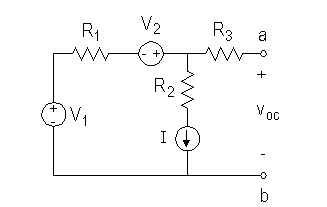# Thevenin Circuit Problem

• Engineering

## Homework StatementV1 = 15 V
V2 = 4 V
I = 2 A
R1 = 3 Ohm
R2 = 4 Ohm
R3 = 8 Ohm
R = 2 Ohm
In this part of the question, I have to solve for the voltage across a and b
R isn't listed as a resistor in this circuit because that comes in a later part of the question (which I can do).

KCL and KVL?

## The Attempt at a Solution

My initial thought was to do KVL across the entire circuit. So that means what I did was I had
-V1 - V2 + (R1 + R2) * I
However, this proved to be wrong
Then I tried to do voltage splitting on the resistors. Which means
[R2 / (R1 + R2)] * (V1 + V2)
This is also wrong

How do I do this question?

I guess either KVL or KCL would do if done correctly.
But the current through R1 is I only if there is no current through R3.
Draw in R. I guess it goes between a and b.
You can get he voltage across R2 and generator I is readily.
This gives the current through R1.
Now you can calculate current through R3+R.

The reason that your KVL equation is not working is because it does not account for the voltage drop across the current source. An ideal current source can have any voltage across it and supply a constant current, 2A in this problem.

An more convenient way to solve the problem is to apply KCL at the node connecting V2, R3, and R2. Let's call this node, node A. Since R3 is floating due to the open circuit the voltage at node A is equal to Voc. The current through R1 is given by the following equation:

$$\begin{center}I_{R_1}=\frac{V_1-(V_{oc}-V_2)}{R_1}\end{center}$$​

We know this current is equal to the current generated by the current source. Just set the above equation equal to the current source current and solve for Voc.

cepheid
Staff Emeritus
Gold Member
EDIT: Nevermind. There is no inconsistency below. One of the two answers I got is wrong because I assumed that the voltage drop across the current source was zero. As klouchis has pointed out, this is incorrect. One of my calculations (the second one I did) agrees with his suggested method for finding Voc. So, ignore this first paragraph below:

I think that the given values might be mutually inconsistent (i.e. the current demanded by the current source is not the same as the current demanded by the other branch given the resistors voltage sources present). Here are my arguments:

I think the easiest way to find the open circuit voltage is to take note of two things:

1. The voltage at both ends of R3 is the same, because there is no current across R3. Therefore, if you consider the "node" that is at the other end of R3 (the end opposite to point a), you will see that this "node" must also be at voltage Voc.

2. The voltage at this node can easily be determined by noticing that the branch with R2 must have current I going through it. The current source demands it. It follows from Ohm's law for that branch that:

Voc = IR2 = (2 A)(4 Ω) = 8 V (EDIT: WRONG because the current source has some non-zero voltage drop across it!!! See method below instead!)

YET, if you look at what the current must be in the other branch (the one with the two voltage sources), you get an inconsistency. KCL would seem to demand that the current in this branch is also I. Ohm's law says that the voltage across R1 is given by the current times the resistance. The voltage across R1 can be calculated as follows. Call the "downstream" side of R1 point 'c.' Then:

Vc + V2 = Voc

OR

Vc = Voc - V2

Ohm's law says:

I = (V1 - Vc)/(R1)

Substituting the expression for Vc:

I = (V1 - Voc + V2)/(R1)

(2 A)(3 Ω) = 15 V - Voc + 4 V

Voc = 15 V + 4 V - 6 V = 13 V.

This is clearly inconsistent with the above answer. (EDIT: That's because the above answer was WRONG).

Last edited:
Cepheid,